• Introduction
• What is in this manual
• What is Caspoc
• User interface
• Introduction
• Starting
• Simulation
• Editing
• Viewing and printing
• Getting Started
• Basic editing
• Simulation in the time domain
• Basic User Interface Topics
• Editing
• Simulation
• Viewing
• Library
• Reports
• Project management
• Circuit and Block Diagram Components
• Introduction
• Cscript and user defined functions
• Component parameters
• Modeling Topics
• Introduction
• Power Electronics
• Semiconductors
• Electrical Machines
• Electrical drives
• Power Systems
• Mechanical Systems
• Thermal Systems
• Magnetic Circuits
• Green Energy
• Coupling to FEM
• Experimenter
• Analog hardware description language
• Embedded C code Export
• Coupling to Spice
• Small Signal Analysis
• Matlab coupling
• Tips and tricks
• Appendices

## Create your first system simulation

In the previous tutorial you created an electric circuit. In this tutorial we will create a system simulation. This means that we will not use any circuit components, but only use system blocks and build a block diagram. For the sake of simplicity we will create a block diagram that will model the theorem of Pythagoras.

For a right angled triangle the square on the hypotenuse is equal to the sum of the squares on the other sides.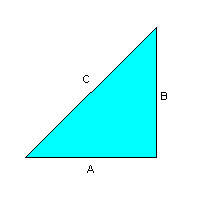A2+B2=C2

We start with opening Caspoc from the start menu. You will see the white work-screen where we will place the components and on the left side the Project Manager from where we will select components. From the Project Manager we will not only select our components, but we can also start tools and read data from it.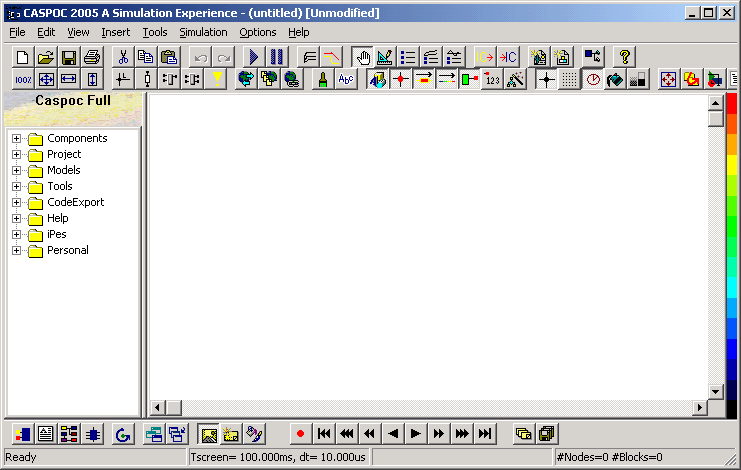On the top of the window you see the menu items as well as many buttons. These buttons are shortcuts to the command that you can select from the menu.

In this tutorial we will create a block diagram for solving c from the above theorem and therefore we select our components from the Project Manager on the left side. Click on the [+] sign in front of Components or double click the item Components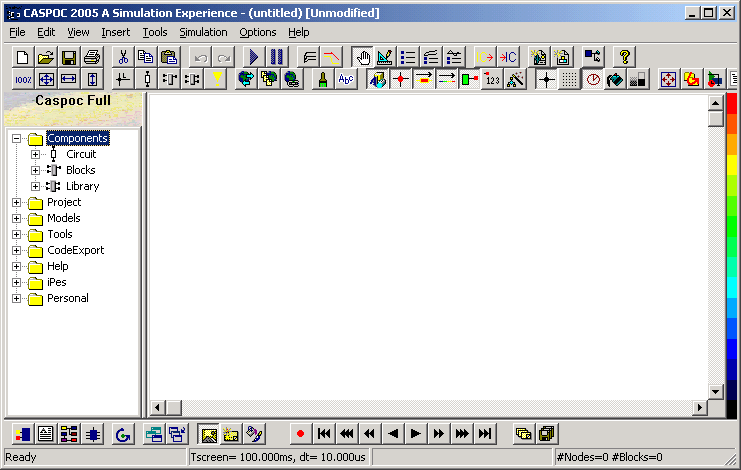The component group will open in the Project Manager and list three sub groups:
• Circuit
• Blocks
• Library
Circuit
From the item Components/Circuit we will select all components that have to do with physical components, for example resistors, inductors, semiconductors, etc. Blocks
From the item Components/Blocks we will select all components that have to do with signals and mathematical relations, for example addition, sinusoidal signals, integrators, etc. Library
From the item Components/Library we will select all components that have to do with a combination of physical components and signals or mathematical relations, for example electrical machines, PI controller, harmonics calculation, etc.

In this simulation we will create a block diagram using multipliers, adders anda square root block. In the Project Manager open the item Components/blocks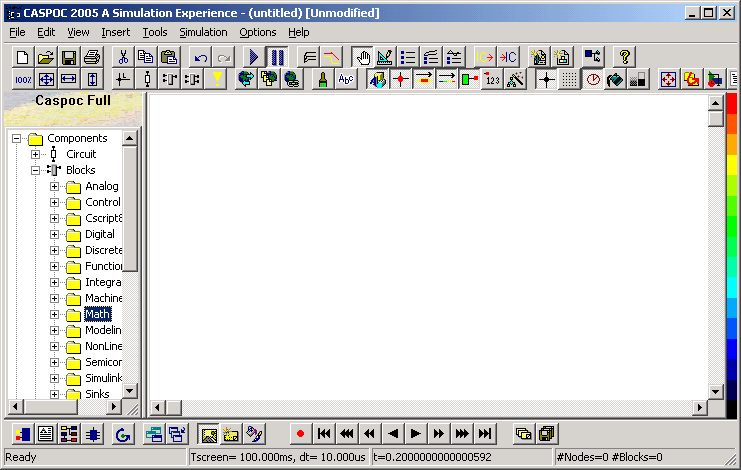It shows you an overview of the categories that contain block diagram components. We will first place a multiplier on the work-screen. To do so, select the item Components/Blocks/Math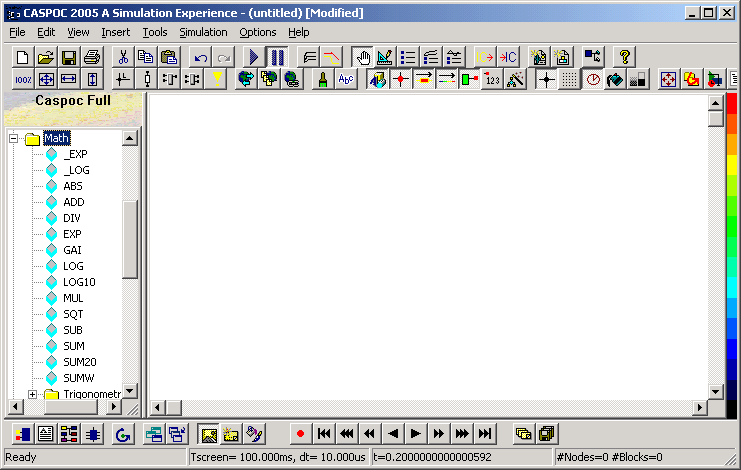You see a list of blocks that can be used in your block diagram. Since we need the square of a and the square of b, we select the block MUL, being a multiplier. You select a component and place it on the screen using the following steps:
1. Move the mouse pointer over the component to be selected, in our case the block MUL
2. Click and release the left mouse button, while the mouse pointer is positioned over the component. It is important to release the mouse button, the component is now sticked to your mouse pointer
3. Drag the mouse pointer over your work-screen to the place where you want the block MUL to be placed.
4. During dragging you will see that the block is sticked to your mouse pointer
5. To place the component, click and release the left mouse button. The component will be dropped onto the work-screen.
The block MUL is now placed on your work-screen.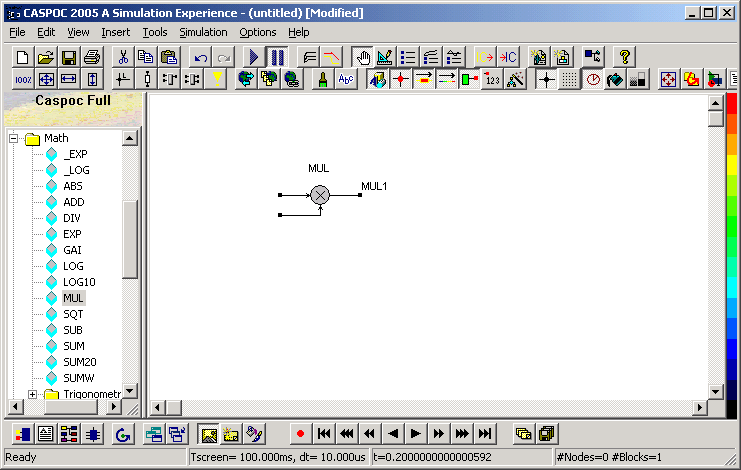The next step is to add the second multiplier to create the quare of b.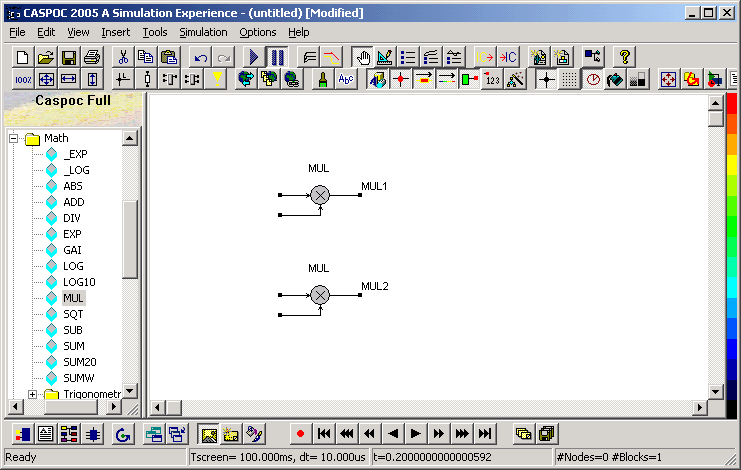We have to add both squares to take the aquare root from. Both signals are added using an ADD block. Select the ADD block from the Project Manager and place it behind both multiplier blocks.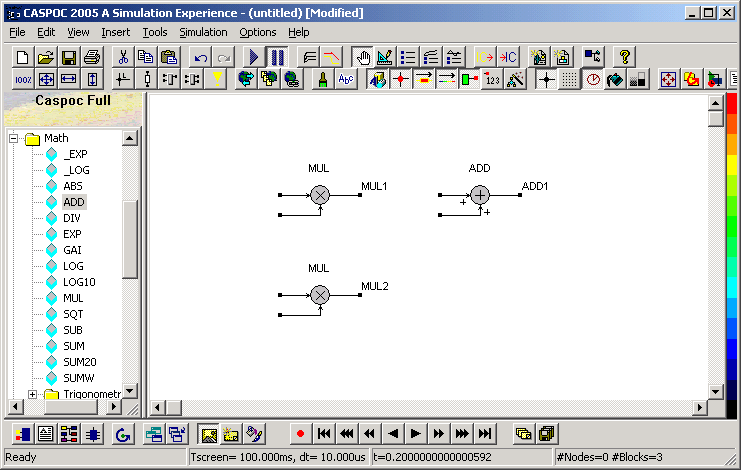From the sum we have to take the square root. Select the block SQT from the Project Manager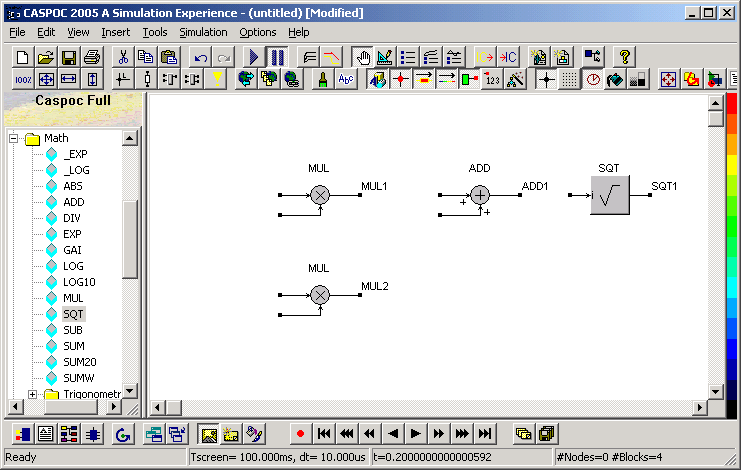All blocks are now placed on the work-screen and we have to connect them. Connections are made by drawing wires between the inputs and outputs of the blocks. The drawing of a wire is started by clicking and releasing the node with the left mouse button. Drag the mouse pointer to the end node and click and release the mouse pointer to connect the wire.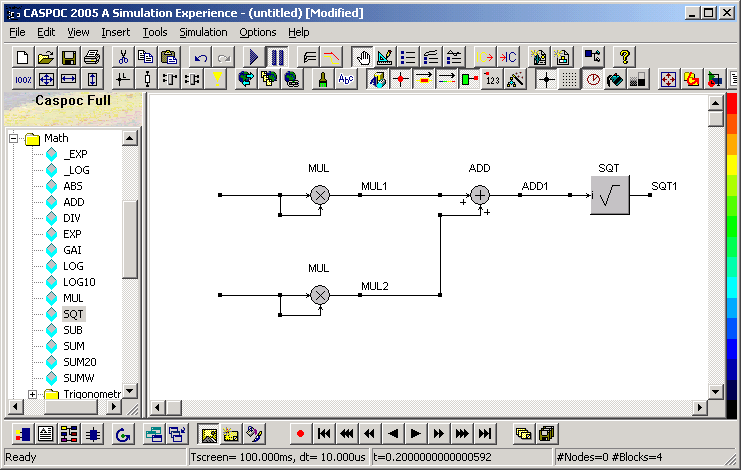Since we would like to input both a and b as numerical values, both inputs of each multiplier are connected by a wire. I you assign a numerical value to a node, it is used as numerical signal in the simulation. We would like to calculate the hypotenuse of a triangle with sides having a length of 3 and 4. This means that the inputs of the multiplier blocks MUL have to get the value 3 and 4 respectively. You assign a numerical value to a node by clicking with the right mouse pointer over the node. Move the mouse pointer over the first node and click the right mouse button.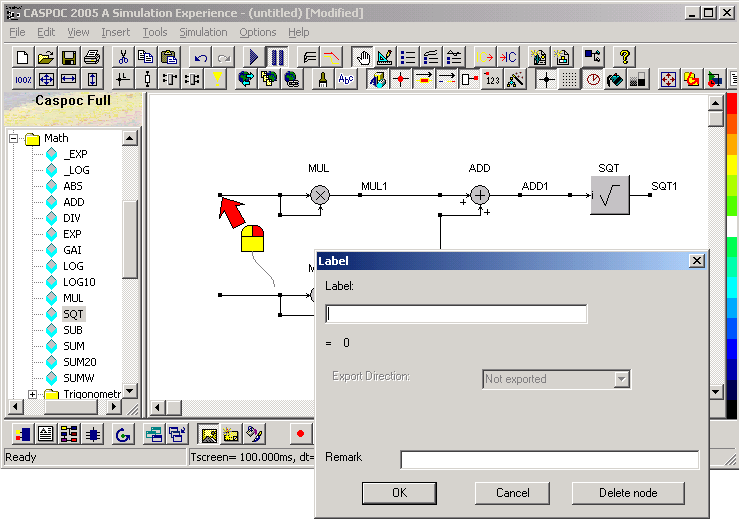Enter the numerical value 3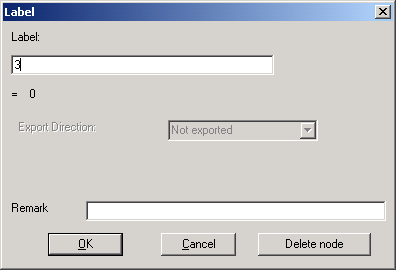and select [OK] to close the label dialog box. Repeat the same for the second input, but now with the numerical value 4.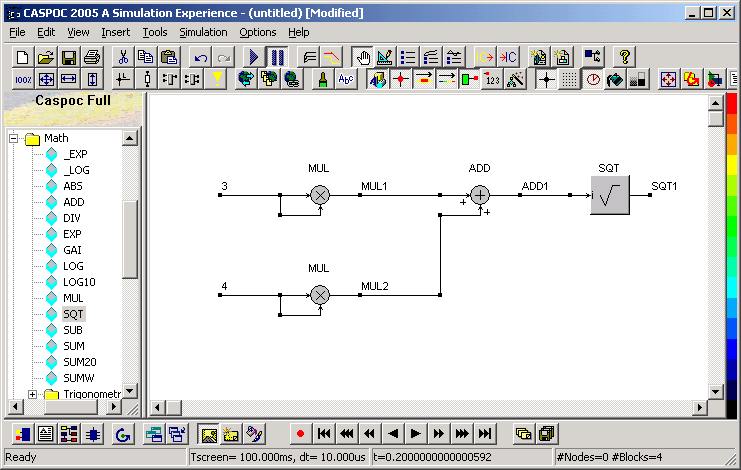Before we start the simulation, be sure that all animation options are enabled. Simply take care that all buttons are enabled as shown in the following button bar.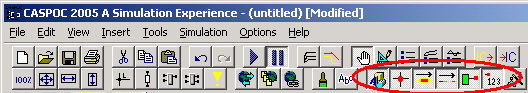Start the simulation by pressing the Play button or pressing the [Enter] key. The simulation is now started and the numerical calulated values of each node will be shown.